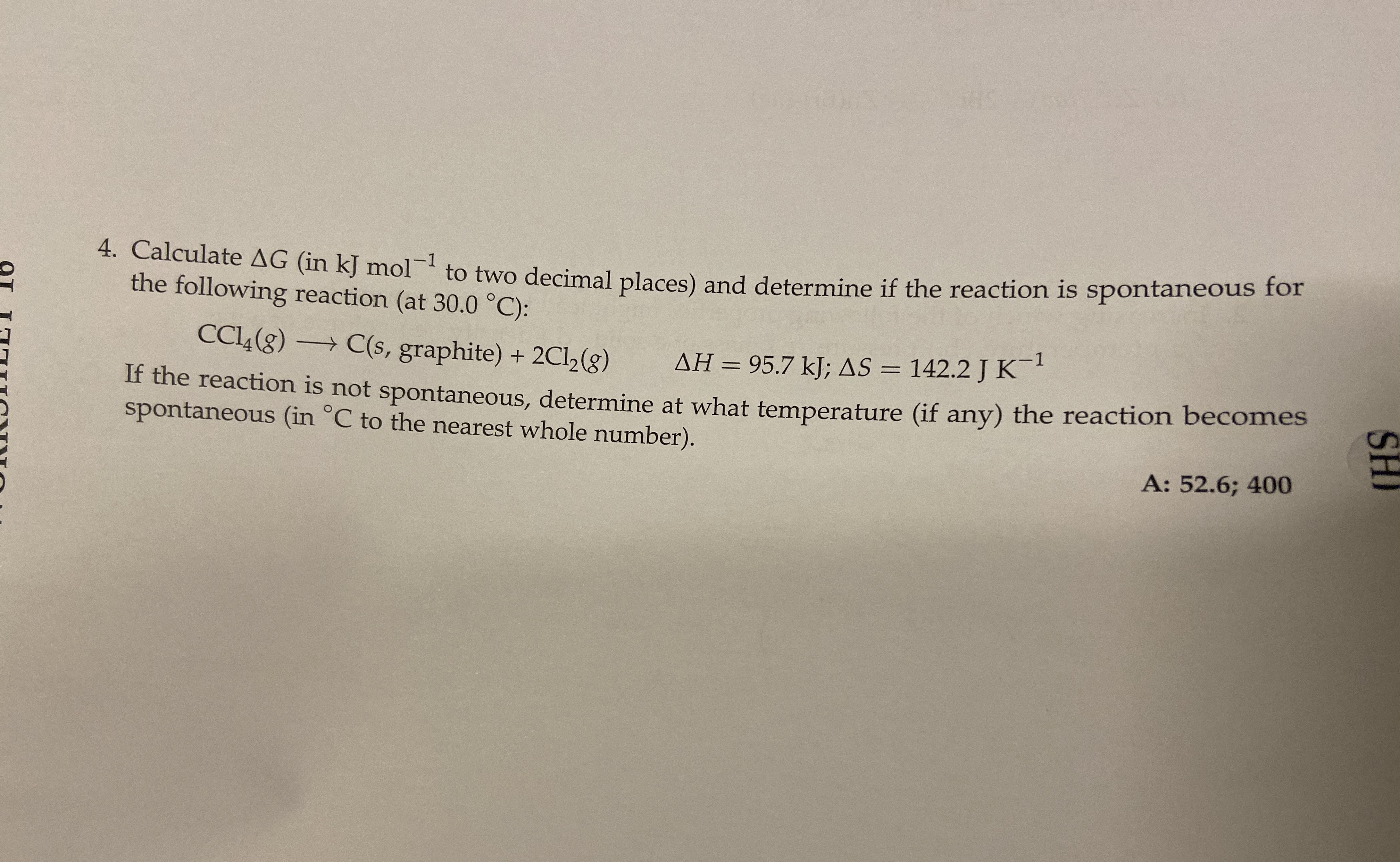# Calculate ΔG (in kJ mol^-1 to two decimal places) and determine if the reaction is spontaneous for the following reaction (at 30 °C): CCl4(g) → C(s, graphite) + 2 Cl2(g) ΔH = 95.7 kJ; ΔS = 142.2 J K^-1 If the reaction is not spontaneous, determine at what temperature (if any) the reaction becomes spontaneous (in °C to the nearest whole number).